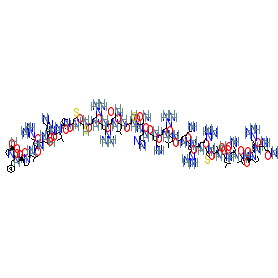#### GtoPdb Ligand ID: 8524

Abbreviated name: ELA-32
Comment: Elabela/Toddler-32 is a predicted dibasic cleavage site isoform of apelin receptor early endogenous ligand
Species: Human2D StructureSMILES / InChI / InChIKeyCanonical SMILES NCCCCC(C(=O)NC(C(=O)NC(C(=O)NC(C(=O)NC(C(=O)NC(C(=O)NC(C(=O)NC(C(=O)NC(C(=O)NC(C(=O)N1CCCC1C(=O)NC(C(=O)NC(C(=O)NC(C(=O)NC(C(=O)NC(C(=O)N1CCCC1C(=O)NC(C(=O)N1CCCC1C(=O)O)Cc1ccccc1)C(C)C)CCCN=C(N)N)CO)Cc1nc[nH]c1)CC(C)C)CCSC)CS)CCCN=C(N)N)CCCN=C(N)N)CCC(=O)N)CC(C)C)CS)CC(=O)N)Cc1nc[nH]c1)NC(=O)C(NC(=O)C(NC(=O)C(NC(=O)C(NC(=O)C(NC(=O)C(NC(=O)C(C(O)C)NC(=O)C(NC(=O)C(NC(=O)C(C(C)C)NC(=O)C1CCCN1C(=O)C(NC(=O)C(CCC(=O)N)N)CCCN=C(N)N)CC(=O)N)CC(C)C)CCSC)CCCN=C(N)N)CCCN=C(N)N)CCCCN)CC(C)C)CCCN=C(N)N Isomeric SMILES NCCCC[C@@H](C(=O)N[C@H](C(=O)N[C@H](C(=O)N[C@H](C(=O)N[C@H](C(=O)N[C@H](C(=O)N[C@H](C(=O)N[C@H](C(=O)N[C@H](C(=O)N[C@H](C(=O)N1CCC[C@H]1C(=O)N[C@H](C(=O)N[C@H](C(=O)N[C@H](C(=O)N[C@H](C(=O)N[C@H](C(=O)N1CCC[C@H]1C(=O)N[C@H](C(=O)N1CCC[C@H]1C(=O)O)Cc1ccccc1)C(C)C)CCCN=C(N)N)CO)Cc1nc[nH]c1)CC(C)C)CCSC)CS)CCCN=C(N)N)CCCN=C(N)N)CCC(=O)N)CC(C)C)CS)CC(=O)N)Cc1nc[nH]c1)NC(=O)[C@@H](NC(=O)[C@@H](NC(=O)[C@@H](NC(=O)[C@@H](NC(=O)[C@@H](NC(=O)[C@@H](NC(=O)[C@H]([C@H](O)C)NC(=O)[C@@H](NC(=O)[C@@H](NC(=O)[C@H](C(C)C)NC(=O)[C@@H]1CCCN1C(=O)[C@@H](NC(=O)[C@H](CCC(=O)N)N)CCCN=C(N)N)CC(=O)N)CC(C)C)CCSC)CCCN=C(N)N)CCCN=C(N)N)CCCCN)CC(C)C)CCCN=C(N)N InChI InChI=1S/C170H291N63O39S4/c1-86(2)70-109(215-137(245)97(36-19-21-55-171)203-133(241)99(38-23-57-194-164(178)179)205-135(243)101(40-25-59-196-166(182)183)208-141(249)106(53-68-275-14)212-158(266)131(92(13)235)229-150(258)112(73-89(7)8)216-148(256)116(78-128(177)239)222-157(265)129(90(9)10)227-156(264)123-47-31-65-231(123)159(267)107(44-29-63-200-170(190)191)213-132(240)96(173)49-51-125(174)236)143(251)209-102(41-26-60-197-167(184)185)136(244)204-98(37-20-22-56-172)138(246)218-113(75-94-79-192-84-201-94)146(254)220-115(77-127(176)238)149(257)226-120(83-274)153(261)217-110(71-87(3)4)144(252)211-105(50-52-126(175)237)140(248)207-100(39-24-58-195-165(180)181)134(242)206-103(42-27-61-198-168(186)187)139(247)225-119(82-273)152(260)214-108(54-69-276-15)160(268)230-64-30-45-121(230)154(262)221-111(72-88(5)6)145(253)219-114(76-95-80-193-85-202-95)147(255)224-118(81-234)151(259)210-104(43-28-62-199-169(188)189)142(250)228-130(91(11)12)162(270)232-66-32-46-122(232)155(263)223-117(74-93-34-17-16-18-35-93)161(269)233-67-33-48-124(233)163(271)272/h16-18,34-35,79-80,84-92,96-124,129-131,234-235,273-274H,19-33,36-78,81-83,171-173H2,1-15H3,(H2,174,236)(H2,175,237)(H2,176,238)(H2,177,239)(H,192,201)(H,193,202)(H,203,241)(H,204,244)(H,205,243)(H,206,242)(H,207,248)(H,208,249)(H,209,251)(H,210,259)(H,211,252)(H,212,266)(H,213,240)(H,214,260)(H,215,245)(H,216,256)(H,217,261)(H,218,246)(H,219,253)(H,220,254)(H,221,262)(H,222,265)(H,223,263)(H,224,255)(H,225,247)(H,226,257)(H,227,264)(H,228,250)(H,229,258)(H,271,272)(H4,178,179,194)(H4,180,181,195)(H4,182,183,196)(H4,184,185,197)(H4,186,187,198)(H4,188,189,199)(H4,190,191,200)/t92-,96+,97+,98+,99+,100+,101+,102+,103+,104+,105+,106+,107+,108+,109+,110+,111+,112+,113+,114+,115+,116+,117+,118+,119+,120+,121+,122+,123+,124+,129+,130+,131+/m1/s1 InChI Key AXGBCVTUKUUUPN-PXWSXZDTSA-N
 References 1. Yang P, Maguire JJ, Kuc RE, Siew K, Haris L, Torella R, Glen RC, Davenport AP.  ELABELA/Toddler, a critical regulator of cardiac development, is expressed in the human cardiovascular system and binds the apelin receptor. Accessed on 07/07/2015. Modified on 07/07/2015. http://circ.ahajournals.org/content/130/Suppl_2/A15352